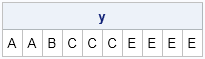A few years ago I blogged about how to expand a data set by using a frequency variable. The DATA step in the article was simple, but the SAS/IML function was somewhat complicated and used a DO loop to expand the data. (Although a reader later showed how to avoid the DO loop.) Consequently, I am happy that the REPEAT function in SAS/IML 12.3 (which shipped with SAS 9.4) supports expanding data by frequency values. Goodbye, complicated function!

To make the situation clear, here is an example from my earlier blog post:

```proc iml; values={A B C D E}; /* categories */ freq = {2 1 3 0 4}; /* nonnegative frequencies */```

The vector values contains five unique categories. The vector freq is the same size and contains the frequency of each category. The goal is to expand the categories by the frequencies to create a new vector that has 10 (sum(freq)) elements. The new vector should contain two copies of 'A', one copy of 'B', three copies of 'C', no copies of 'D', and four copies of 'E'. The new syntax for the REPEAT function makes this easy:

```y = repeat(values, freq); /* SAS/IML 12.3 */ print y;```The REPEAT function does not support negative or missing values, but you can use the LOC function to remove those invalid values:

```values={A B C D E F G}; freq = {2 1 3 0 4 -2 .}; posIdx = loc(freq>0); /* select positive frequency values */ y = repeat(values[posIdx], freq[posIdx]);```
Share